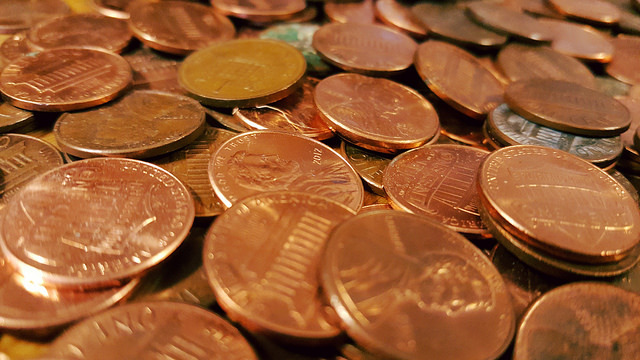# Balance Scale As A Mathematical Model# Which Coin Is Counterfeit?

Suppose you have 12 coins, and one of the coins is counterfeit. You know that the counterfeit coin weighs less than the other coins.

1. Can you use a balance scale to find the counterfeit coin?
2. Turn and talk to your partner about this question. Share an idea about how you might approach the problem, but do not try to find the solution yet.## Hint:

• Counterfeit means fake ; a counterfeit coin looks like a real coin issued by the government but is actually an imitation.
• A counterfeit coin is made from cheaper metals, so it weighs less than a real coin.
1 of 5